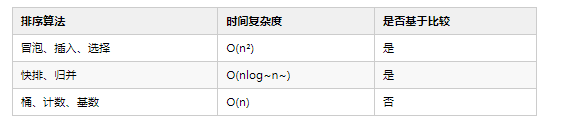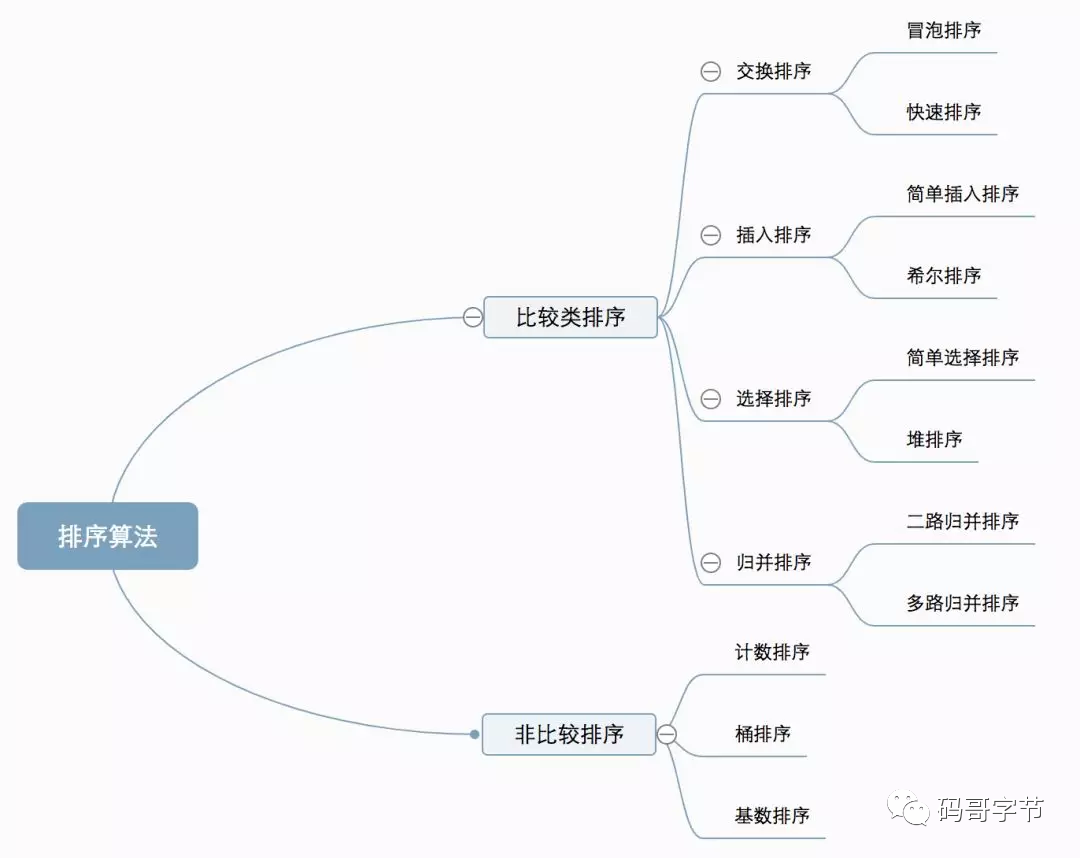# 经典 O(n²)比较类排序算法

0收藏1. 比较类排序：通过比较来决定元素的相对次序，由于其时间复杂度无法突破 O(nlog~n~)，因此也叫做非线性时间排序。1.最好、最坏、平均时间复杂度

2. 时间复杂度的系数、常数、低阶

3.比较次数移动（交换）数据次数基于比较排序的算法执行过程都会涉及两个操作、一个是比较，另一个就是元素交换或者数据移动。所以我们也要把数据交换或者移动次数考虑进来。

1.比较相邻的元素。如果第一个比第二个大，就交换他们两个。
2.对每一对相邻元素作同样的工作，从开始第一对到结尾的最后一对。这步做完后，最后的元素会是最大的数。
3.针对所有的元素重复以上的步骤，除了最后一个。

``````/**
* 冒泡排序: 时间复杂度 O(n²)，最坏时间复杂度 O(n²)，最好时间复杂度 O(n)，平均时间复杂度 O(n²)
* 空间复杂度 O(1)，稳定排序算法
*/
public class BubbleSort implements ComparisonSort {
@Override
public int[] sort(int[] sourceArray) {
// 复制数组，不改变参数内容
int[] result = Arrays.copyOf(sourceArray, sourceArray.length);
if (sourceArray.length <= 1) {
return result;
}
int length = result.length;
for (int i = 0; i < length; i++) {
// 设定标记，当没有数据需要交换的时候则说明已经有序，提前退出外部循环
boolean hasChange = false;
for (int j = 0; j < (length - 1) - i ; j++) {
if (result[j] > result[j + 1]) {
// 数据交换
int temp = result[j];
result[j] = result[j + 1];
result[j + 1] = temp;
hasChange = true;
}
}
if (!hasChange) {
// 没有数据交换，已经有序,提前退出
break;
}
}
return result;
}
}
``````

1.冒泡是原地排序算法么？

2.是稳定排序算法？

3.时间复杂度

``````/**
* 插入排序：时间复杂度 O(n²),平均时间复杂度 O(n²),最好时间复杂度 O(n)，
* 最坏时间复杂度 O(n²),空间时间复杂度 O(1).稳定排序算法。
*/
public class InsertionSort implements ComparisonSort {

@Override
public int[] sort(int[] sourceArray) {
int[] result = Arrays.copyOf(sourceArray, sourceArray.length);
if (sourceArray.length <= 1) {
return result;
}
// 从下标为 1 开始比较选择合适位置插入，因为下标 0 只有一个元素，默认是有序
int length = result.length;
for (int i = 1; i < length; i++) {
// 待插入数据
int insertValue = result[i];
// 从已排序的序列最右边元素开始比较，找到比待插入树更小的数位置
int j = i - 1;
for (; j >= 0; j--){
if (result[j] > insertValue) {
// 向后移动数据,腾出待插入位置
result[j + 1] = result[j];
} else {
// 找到待插入位置，跳出循环
break;
}
}
// 插入数据，因为前面多执行了 j--，
result[j + 1] = insertValue;
}
return result;
}
}
``````

1.是否是原地排序算法

2.是否是稳定排序算法

3.时间复杂度

1.首先在未排序序列中找到最小（大）元素，存放到排序序列的起始位置
2.再从剩余未排序元素中继续寻找最小（大）元素，然后放到已排序序列的末尾。
3.重复第二步，直到所有元素均排序完毕。

``````public class SelectionSort implements ComparisonSort {

@Override
public int[] sort(int[] sourceArray) {
int length = sourceArray.length;
int[] result = Arrays.copyOf(sourceArray, length);
if (length <= 0) {
return result;
}
// 一共需要 length - 1 轮比较
for (int i = 0; i < length - 1; i++) {
// 每轮需要比较的次数 length - i，找出最小元素下标
int minIndex = i;
for (int j = i + 1; j < length; j++) {
if (result[j] < result[minIndex]) {
// 查出每次最小元素下标
minIndex = j;
}
}
// 将当前 i 位置的数据与最小值交换数据
if (i != minIndex) {
int temp = result[i];
result[i] = result[minIndex];
result[minIndex] = temp;
}
}
return result;
}
}
``````社区精华内容